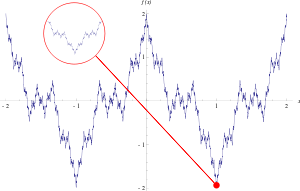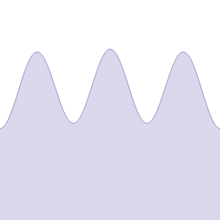# Weierstrass function

Not to be confused with the Weierstrass elliptic function () or the Weierstrass sigma, zeta, or eta functions.Plot of Weierstrass function over the interval [2, 2]. Like fractals, the function exhibits self-similarity: every zoom (red circle) is similar to the global plot.

In mathematics, the Weierstrass function is an example of a pathological real-valued function on the real line. The function has the property of being continuous everywhere but differentiable nowhere. It is named after its discoverer Karl Weierstrass.

Historically, the Weierstrass function is important because it was the first published example (1872) to challenge the notion that every continuous function was differentiable except on a set of isolated points.

## ConstructionAnimation based on the increasing of the b value from 0.1 to 5.

In Weierstrass' original paper, the function was defined as the sum of a Fourier series:where,is a positive odd integer, andThe minimum value ofwhich satisfies these constraints is. This construction, along with the proof that the function is nowhere differentiable, was first given by Weierstrass in a paper presented to the Königliche Akademie der Wissenschaften on 18 July 1872.

The proof that this function is continuous everywhere is not difficult. Since the terms of the infinite series which defines it are bounded by ±an and this has finite sum for 0 < a < 1, convergence of the sum of the terms is uniform by the Weierstrass M-test with Mn = an. Since each partial sum is continuous, by the uniform limit theorem, it follows that f is continuous. Additionally, since each partial sum is uniformly continuous, it follows that f is also uniformly continuous.

Naïvely it might be expected that a continuous function must have a derivative, or that the set of points where it is not differentiable should be "small" in some sense. According to Weierstrass in his paper, earlier mathematicians including Gauss had often assumed that this was true. This might be because it is difficult to draw or visualise a continuous function whose set of nondifferentiable points is something other than a countable set of points. Analogous results for better behaved classes of continuous functions do exist, for example the Lipschitz functions, whose set of non-differentiability points must be a Lebesgue null set (Rademacher's theorem). When we try to draw a general continuous function, we usually draw the graph of a function which is Lipschitz or otherwise well-behaved.

The Weierstrass function could perhaps be described as one of the very first fractals studied, although this term was not used until much later. The function has detail at every level, so zooming in on a piece of the curve does not show it getting progressively closer and closer to a straight line. Rather between any two points no matter how close, the function will not be monotone. The Hausdorff dimension D of the graph of the classical Weierstrass function is bounded above by 2 + ln(a)/ln(b), (where a and b are the constants in the construction above) and is generally believed to be exactly that value, but this had not been proven rigorously. Notice that 1 < D < 2 if ab > 1.

The term Weierstrass function is often used in real analysis to refer to any function with similar properties and construction to Weierstrass' original example. For example, the cosine function can be replaced in the infinite series by a piecewise linear "zigzag" function. G. H. Hardy showed that the function of the above construction is nowhere differentiable with the assumptions 0 < a < 1, ab ≥ 1.

## Hölder continuity

It is convenient to write the Weierstrass function equivalently asfor. Then Wα(x) is Hölder continuous of exponent α, which is to say that there is a constant C such thatfor all x and y. Moreover, Wα is Hölder continuous of all orders α < 1 but not Lipschitz continuous.

## Density of nowhere-differentiable functions

It turns out that the Weierstrass function is far from being an isolated example: although it is "pathological", it is also "typical" of continuous functions:

• In a topological sense: the set of nowhere-differentiable real-valued functions on [0, 1] is comeager in the vector space C([0, 1]; R) of all continuous real-valued functions on [0, 1] with the topology of uniform convergence.
• In a measure-theoretic sense: when the space C([0, 1]; R) is equipped with classical Wiener measure γ, the collection of functions that are differentiable at even a single point of [0, 1] has γ-measure zero. The same is true even if one takes finite-dimensional "slices" of C([0, 1]; R), in the sense that the nowhere-differentiable functions form a prevalent subset of C([0, 1]; R).

## Notes

1. At least two researchers formulated continuous, nowhere differentiable functions before Weierstrass, but their findings were not published in their lifetimes. Around 1831, Bernard Bolzano (1781 - 1848), a Czech mathematician, philosopher, and Catholic priest, constructed such a function; however, it was not published until 1922. See:
• Martin Jašek (1922) "Funkce Bolzanova" (Bolzano's function), Časopis pro Pěstování Matematiky a Fyziky (Journal for the Cultivation of Mathematics and Physics), vol. 51, no. 2, pages 69 - 76 (in Czech and German).
• Vojtěch Jarník (1922) "O funkci Bolzanově" (On Bolzano's function), Časopis pro Pěstování Matematiky a Fyziky (Journal for the Cultivation of Mathematics and Physics), vol. 51, no. 4, pages 248 - 264 (in Czech). Available on-line in Czech at: http://dml.cz/bitstream/handle/10338.dmlcz/109021/CasPestMatFys_051-1922-4_5.pdf . Available on-line in English at: http://dml.cz/bitstream/handle/10338.dmlcz/400073/Bolzano_15-1981-1_6.pdf .
• Karel Rychlík (1923) "Über eine Funktion aus Bolzanos handschriftlichem Nachlasse" (On a function from Bolzano's literary remains in manuscript), Sitzungsberichte der königlichen Böhmischen Gesellschaft der Wissenschaften (Prag) (Proceedings of the Royal Bohemian Society of Philosophy in Prague) (for the years 1921-1922), Class II, no. 4, pages 1-20. (Sitzungsberichte was continued as: Věstník Královské české společnosti nauk, třída matematicko-přírodovědecká (Journal of the Royal Czech Society of Science, Mathematics and Natural Sciences Class).)
Around 1860, Charles Cellérier (1818 - 1889), a professor of mathematics, mechanics, astronomy, and physical geography at the University of Geneva, Switzerland, independently formulated a continuous, nowhere differentiable function that closely resembles Weierstrass's function. Cellérier's discovery was, however, published posthumously:
2. On page 560 of the 1872 Monatsberichte der Königlich Preussischen Akademie der Wissenschaften zu Berlin (Monthly Reports of the Royal Prussian Academy of Science in Berlin), there is a brief mention that on July 18th, "Hr. Weierstrass las über stetige Funktionen ohne bestimmte Differentialquotienten" (Mr. Weierstrass read [a paper] about continuous functions without definite [i.e., well-defined] derivatives [to members of the Academy]). However, Weierstrass's paper was not published in the Monatsberichte.
3. Karl Weierstrass, "Über continuirliche Functionen eines reellen Arguments, die für keinen Werth des letzeren einen bestimmten Differentialquotienten besitzen," (On continuous functions of a real argument which possess a definite derivative for no value of the argument) in: Königlich Preussichen Akademie der Wissenschaften, Mathematische Werke von Karl Weierstrass (Berlin, Germany: Mayer & Mueller, 1895), vol. 2, pages 71–74.;
4. See also: Karl Weierstrass, Abhandlungen aus der Functionenlehre [Treatises from the Theory of Functions] (Berlin, Germany: Julius Springer, 1886), page 97.
5. Kenneth Falconer,The Geometry of Fractal Sets (Cambridge, England: Cambridge University Press, 1985), pages 114, 149.
6. See also: Brian R. Hunt (1998) "The Hausdorff dimension of graphs of Weierstrass functions," Proceedings of the American Mathematical Society, vol. 126, no. 3, pages 791-800.
7. Hardy G. H. (1916) "Weierstrass's nondifferentiable function," Transactions of the American Mathematical Society, vol. 17, pages 301–325.
8. Zygmund, A. (2002) , Trigonometric series. Vol. I, II, Cambridge Mathematical Library (3rd ed.), Cambridge University Press, ISBN 978-0-521-89053-3, MR 1963498, p. 47.
9. Mazurkiewicz, S.. (1931). "Sur les fonctions non dérivables". Studia. Math. (3): 92–94.
10. Banach, S. (1931). "Über die Baire'sche Kategorie gewisser Funktionenmengen". Studia. Math. (3): 174–179.

## References

• B.R. Gelbaum and J.M.H. Olmstead, Counterexamples in Analysis, Holden Day Publisher (June 1964).
• Karl Weierstrass, "Über continuirliche Functionen eines reellen Arguments, die für keinen Werth des letzeren einen bestimmten Differentialquotienten besitzen," in: Königlich Preussichen Akademie der Wissenschaften, Mathematische Werke von Karl Weierstrass (Berlin, Germany: Mayer & Mueller, 1895), vol. 2, pages 71–74.; English translation: On continuous functions of a real argument that do not possess a well-defined derivative for any value of their argument, in: G.A. Edgar, Classics on Fractals, Addison-Wesley Publishing Company, 1993, 3–9.
• G.H. Hardy, "Weierstrass's nondifferentiable function," Trans. Amer. Math. Soc., 17(1916), 301–325.
• K. Falconer, The Geometry of Fractal Sets, Oxford (1984).